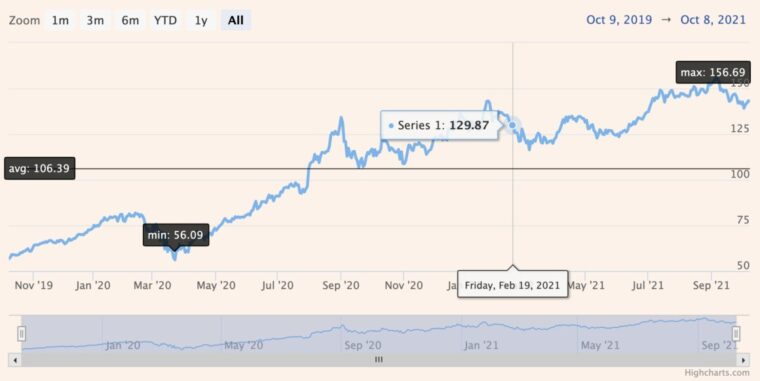# Automated AVG plotLine, min and max labels using annotations (Part 2)Here is another exciting topic of the series of articles about how to use the Highcharts annotation module to display and highlight important information on the chart. This article will focus on dynamically positioning an annotation to calculate its position based on the chart’s and series’ properties.

The goal is to create an annotation, which consists of one shape and 3 labels. As we pointed out in the previous article, the annotations’ configuration is an array of objects, whereas, for each object, we can specify an unlimited number of labels and shapes. In our case, the shape will be a line, which will indicate the average value of the visible series with one label describing its value, and two additional labels to specify the current min and max values.

Our initial chart configuration looks like this:

```Highcharts.getJSON('https://demo-live-data.highcharts.com/aapl-c.json', function(data) {
Highcharts.stockChart('container', {
chart: {
zoomType: 'x'
},
xAxis: {
type: 'datetime'
},
series: [{
data: data
}]
});
});```

The first step of creating our annotation is to define its initial configuration:

```annotations: [{
draggable: '',
shapes: [],
labels: []
}],```

We define the `draggable: ‘’` property so that the dragging feature is disabled. We are calculating the labels’ and shape’s positions for correct positioning.
The next step is defining a shape configuration. The shape will be `type: ‘path’` which is the most effective annotation for drawing straight lines. We need to add the points array with at least two points’ positions to draw this annotation. And here is the fun part: The points’ positions don’t have to be added as objects; they can be callback functions, which will return the desired position of the points! Using this piece of information, we can create the shapes config in the following way:

```shapes: [{
type: 'path',
points: [
annotation => {
// Calculate once
getMinMax(annotation.chart);

return {
x: annotation.chart.xAxis.min,
xAxis: 0,
y: (minMax.yMin + minMax.yMax) / 2,
yAxis: 0
};
},
annotation => ({
x: annotation.chart.xAxis.max,
xAxis: 0,
y: (minMax.yMin + minMax.yMax) / 2,
yAxis: 0
})
]
}],```

As you notice, we also call the `getMinMax(annotation.chart);` function, which calculates the current min and max for each axis. Then it assigns calculated values to a global variable (`minMax`), which is accessible from each callback function so that it needs to be called only once.
This function looks like this:

```function getMinMax(chart) {
const yMin = Math.min.apply(null, chart.series.processedYData.slice(1, -1)),
yMax = Math.max.apply(null, chart.series.processedYData.slice(1, -1)),
maxIndex = chart.series.processedYData.indexOf(yMax),
minIndex = chart.series.processedYData.indexOf(yMin);
minMax = {
xMin: chart.series.processedXData[minIndex],
xMax: chart.series.processedXData[maxIndex],
yMin,
yMax
}
}```

As of now, we have the line rendered. Let’s move our focus to labels; their config will be basic as well. Since we have the values calculated, we just need to form the correct value for each label. Each label’s config consists of the point callback function that will return its position. Some other fields are used to describe the label’s format, position relative to the calculated point, and some clipping options.
Here is a configuration of a first label:

```{
point: () => ({
x: minMax.xMax,
xAxis: 0,
y: minMax.yMax,
yAxis: 0
}),
format: 'max: {y:.2f}'
},```

As you can see, the point function is really simple, yet thanks to the fact that it is fired in each render, the label and shape adjust to the current chart and update after some events, like zooming in or panning the current plot area.
Full chart configuration looks like this:

```Highcharts.getJSON(
'https://demo-live-data.highcharts.com/aapl-c.json',
function(data) {
let minMax = {};

function getMinMax(chart) {
const yMin = Math.min.apply(
null,
chart.series.processedYData.slice(1, -1)
),
yMax = Math.max.apply(
null,
chart.series.processedYData.slice(1, -1)
),
maxIndex = chart.series.processedYData.indexOf(yMax),
minIndex = chart.series.processedYData.indexOf(yMin);

minMax = {
xMin: chart.series.processedXData[minIndex],
xMax: chart.series.processedXData[maxIndex],
yMin,
yMax
};
}

Highcharts.stockChart('container', {
chart: {
zoomType: 'x'
},

xAxis: {
type: 'datetime'
},

annotations: [{
draggable: '',
shapes: [{
type: 'path',
points: [
annotation => {
// Calculate once
getMinMax(annotation.chart);

return {
x: annotation.chart.xAxis.min,
xAxis: 0,
y: (minMax.yMin + minMax.yMax) / 2,
yAxis: 0
};
},
annotation => ({
x: annotation.chart.xAxis.max,
xAxis: 0,
y: (minMax.yMin + minMax.yMax) / 2,
yAxis: 0
})
]
}],
labels: [{
point: () => ({
x: minMax.xMax,
xAxis: 0,
y: minMax.yMax,
yAxis: 0
}),
format: 'max: {y:.2f}'
}, {
point: () => ({
x: minMax.xMin,
xAxis: 0,
y: minMax.yMin,
yAxis: 0
}),
format: 'min: {y:.2f}'
}, {
point: annotation => ({
x: annotation.chart.xAxis.min + 10e7,
xAxis: 0,
y: (minMax.yMin + minMax.yMax) / 2,
yAxis: 0
}),
y: 11,
x: 30,
clip: false,
overflow: 'none',
format: 'avg: {y:.2f}'
}]
}],
series: [{
data: data
}]
});
}
);```

It looks pretty basic, right? Here is a live example:

Notice that the labels’ and shape’s position update when you zoom in or change the extremes using the navigator. As you can see, the annotation module is really powerful and allows you to configure the chart in really interesting ways.

Let us know, if you find this article useful, and don’t hesitate to reach out to us in the comments or one of our support channels.